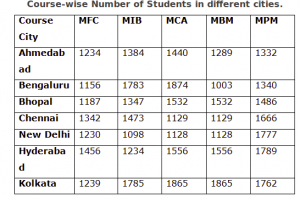## Data Interpretation For SBI PO : Set -31

D.1-5) Study the table carefully to answer the questions that follow:-1) In Chennai, the number of MFC students is approximately what percent of that of MPM students?

a) 69

b) 90

c) 94

d) 73

e) 81

e)

Number of students of MFC in Chennai = 1342

Number of students of MPM in Chennai = 1666

∴ Required percentage

= 1342/1666 x 100 = 80.55 = 81

2) In Ahmedabad, the number of MBM students is approximately what percent of that of MCA students?

a) 66

b) 71

c) 84

d) 87

e) 90

e)

Number of MBM students in Ahmedabad = 1289

Number of MCA students in Ahmedabad = 1440

Required percentage = 1289/1440 x 100

= 89.5 = 90

3) What is the difference between total number of MIB and MFC students from all the cities together?

a) 1140

b) 1260

c) 1330

d) 1480

e) None of these

b)

Total Number of MIB  students in all citites = 10104

Total Number of MFC students in all cities = 8844

Difference 10104 – 8844 = 1260

4) Which City has the highest number of students?

c) Chennai

d) Kolkata

e) None of these

d)

Total number of students :

Bangaluru – 1156 + 1783 + 1874 + 1003 + 1340 = 7156

Chennai – 1342 + 1473 + 1129 + 1765 + 1666 = 7375

New Delhi – 1230 + 1098 + 1128 + 1865 + 1777 = 7098

Hyderabad – 1456 + 1234 + 1556 + 1653 + 1789 = 7688

Kolkota – 1239 + 1785 + 1865 + 1504 + 1762 = 8155

The number of students was highest in Kolkata.

5) What is the approximate average number of students enrolled in MIB course from all the cities together?

a) 1263

b) 1165

c) 1443

d) 1503

e) 1349

c)

Number of students enrolled in MIB course

= 1384 + 1783 + 1347 + 1473 + 1098 = 10104

Required average = 10104/7

= 1443

D.6-10) Study the following table and answer the questions based on it6) The total expenditure of the company over these items during the year 2000 is?

a) Rs.544.44 lakhs

b) Rs.501.11 lakhs

c) Rs.446.46 lakhs

d) Rs.478.87 lakhs

e) None of these

a)

Total expenditure of the Company during 2000 (324 + 101 + 3.84 + 41.6 + 74) lakhs

Rs. 544.44 lakhs.

7) What is the average amount of interest per year which the company had to pay during this period?

a) Rs.32.43 lakhs

b) Rs.33.72 lakhs

c) Rs.34.18 lakhs

d) Rs.36.66 lakhs

e) None of these

d)

Average amount of interest paid by the Company during the given period

23.4 + 32.5 + 41.6 + 36.4 + 49.4 /5 = 36.6 lakhs

8) The total amount of bonus paid by the company during the given period is approximately what percent of the total amount of salary paid during this period?

a) 0.1%

b) 0.5%

c) 1%

d) 1.25%

e) None of these

c)

(3.00 + 2.52 + 3.84 + 3.68 + 3.96) / (288 + 342 + 324 + 336 + 420) *100 = 1%

9) Total expenditure on all these items in 1998 was approximately what percent of the total expenditure in 2002?

a) 62%

b) 66%

c) 69%

d) 71%

e) None of these

c)

(288 + 98 + 3.00 + 23.4 + 83)/(420 + 142 + 3.96 + 49.4 + 98)*100 = 69.45%.

10) The ratio between the total expenditure on taxes for all the years and the total expenditure on fuel and transport for all the years respectively is approximately?

a) 4 : 7

b) 10 : 13

c) 15 : 18

d) 5 : 8

e) None of these